# 林轩田机器学习基石课程学习笔记14 — Regularization

## 一、Regularized Hypothesis Set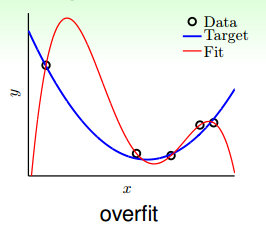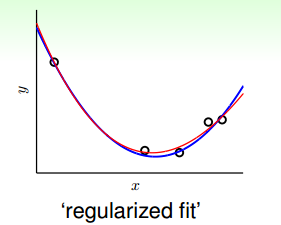H_{10}=w_0+w_1x+w_2x^2+w_3x^3+\cdots+w_{10}x^{10}

H_2可表示为：

H_2=w_0+w_1x+w_2x^2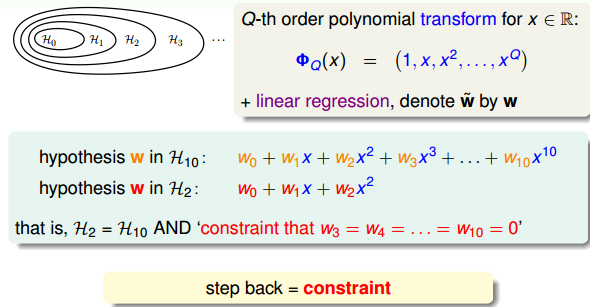\sum_{q=0}^{10}(w_q\neq0)\leq3

H_2\subset H_2’\subset H_{10}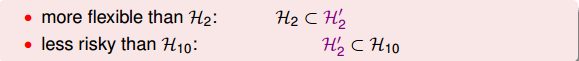Looser Constraint对应的hypothesis应该更好解一些，但事实是sparse hypothesis set H_2′被证明也是NP-hard，求解非常困难。所以，还要转换为另一种易于求解的限定条件。

\sum_{q=0}^{10}w_q^2=||w||^2\leq C

H_2′H(C)的关系是，它们之间有重叠，有交集的部分，但是没有完全包含的关系，也不一定相等。对应H(C)，C值越大，限定的范围越大，即越宽松：

H(0)\subset H(1.126)\subset \cdots \subset H(1126)\subset \cdots \subset H(\infty)=H_{10}

## 二、Weight Decay Regularization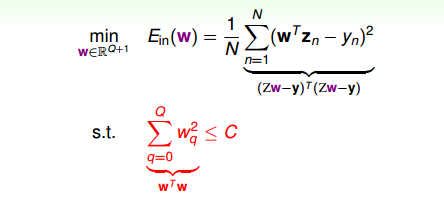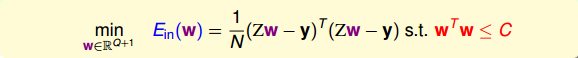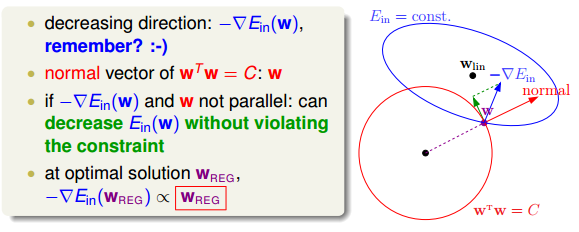\nabla E_{in}(w_{REG})+\frac{2\lambda}{N}w_{REG}=0

E_{in}=\frac1N\sum_{n=1}^N(x_n^Tw-y_n)^2

\frac2N(Z^TZw_{REG}-Z^Ty)+\frac{2\lambda}Nw_{REG}=0

w_{REG}=(Z^TZ+\lambda I)^{-1}Z^Ty

\nabla E_{in}(w_{REG})+\frac{2\lambda}{N}w_{REG}=0

E_{aug}(w)=E_{in}(w)+\frac{\lambda}Nw^Tw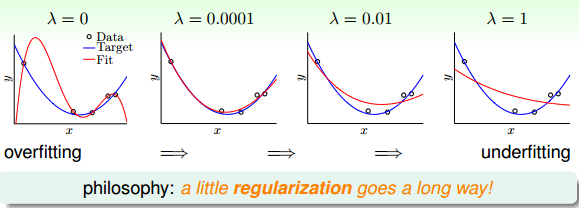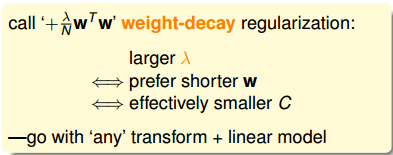## 三、Regularization and VC Theory

E_{aug}(w)=E_{in}(w)+\frac{\lambda}Nw^Tw

VC Bound表示为：

E_{out}(w)\leq E_{in}(w)+\Omega(H)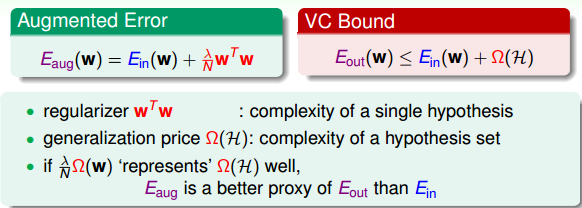d_{EFF}(H,A)\leq d_{VC}

## 四、General Regularizers

• target-dependent

• plausible

• friendly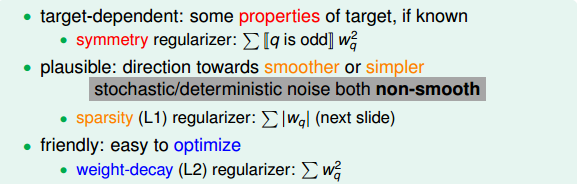• user-dependent

• plausible

• friendly

regularizer与error measure是机器学习模型设计中的重要步骤。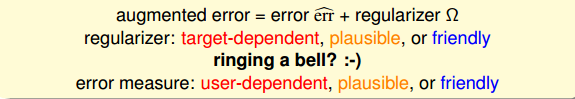\Omega(w)=\sum_{q=0}^Qw_q^2=||w||_2^2

L1 Regularizer的表达式如下：

\Omega(w)=\sum_{q=0}^Q|w_q|=||w||_1

L1计算的不是w的平方和，而是绝对值和，即长度和，也是凸函数。已知w^Tw=C围成的是圆形，而||w||_1=C围成的是正方形，那么在正方形的四个顶点处，是不可微分的（不像圆形，处处可微分）。根据之前介绍的平行等式推导过程，对应这种正方形，它的解大都位于四个顶点处（不太理解，欢迎补充赐教），因为正方形边界处的w绝对值都不为零，若-\nabla E_{in}不与其平行，那么w就会向顶点处移动，顶点处的许多w分量为零，所以，L1 Regularizer的解是稀疏的，称为sparsity。优点是计算速度快。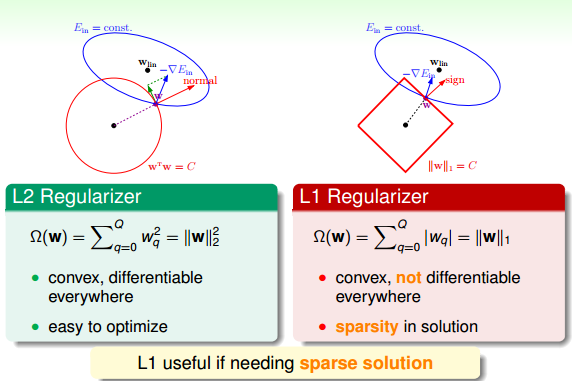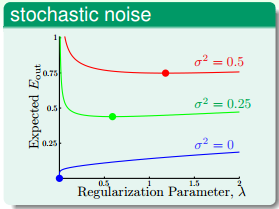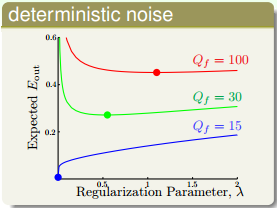## 五、总结### 觉得文章有用就打赏一下文章作者

#### 支付宝扫一扫打赏#### 微信扫一扫打赏